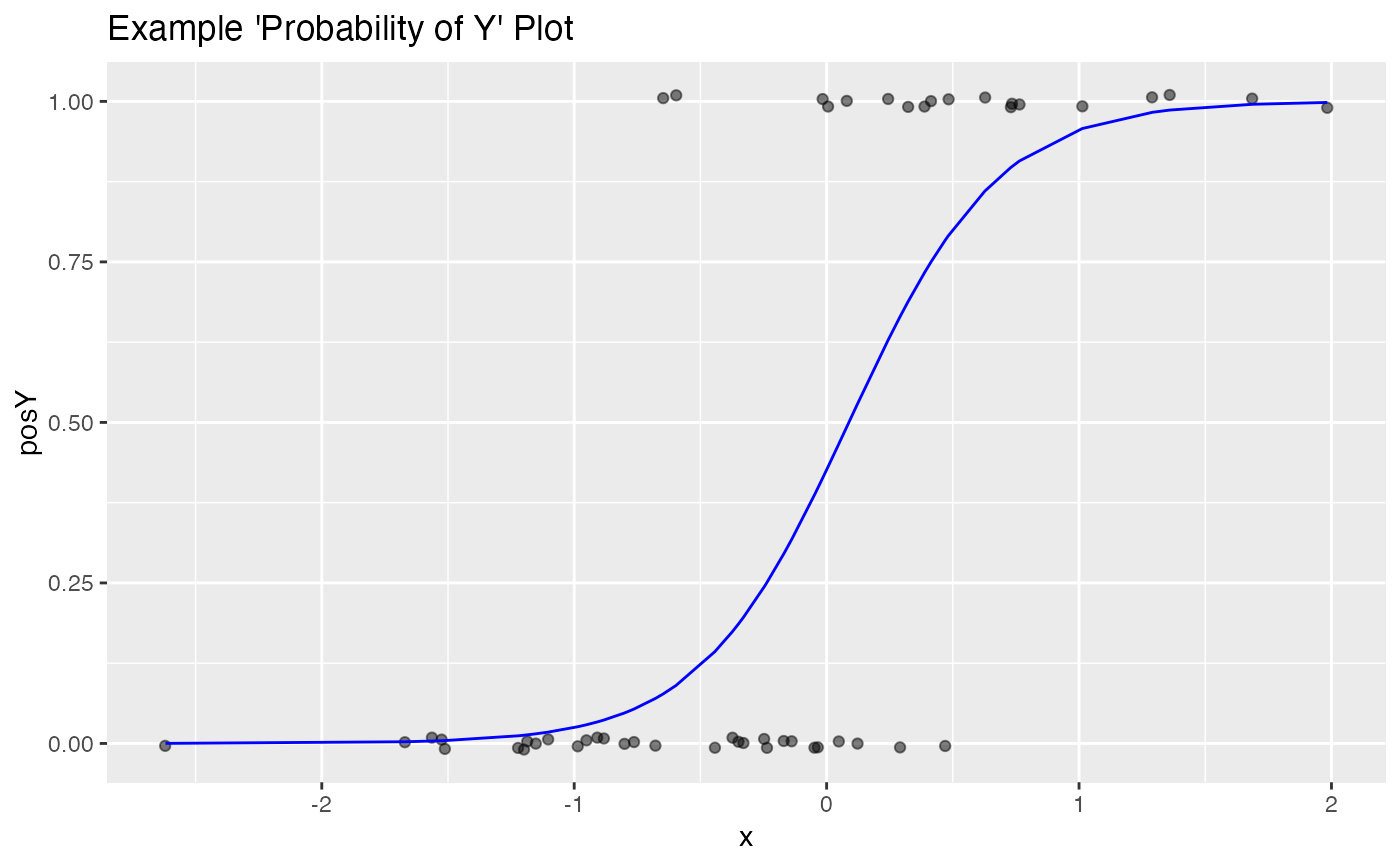Plot the scatter plot of a binary variable with a smoothing curve.

BinaryYScatterPlot(
frame,
xvar,
yvar,
title,
...,
se = FALSE,
use_glm = TRUE,
point_color = "black",
smooth_color = "blue"
)

## Arguments

frame data frame to get values from name of the independent column in frame name of the dependent (output or result to be modeled) column in frame title to place on plot no unnamed argument, added to force named binding of later arguments. if TRUE, add error bars (defaults to FALSE). Ignored if useGLM is TRUE if TRUE, "smooths" with a one-variable logistic regression (defaults to TRUE) color for points color for smoothing line

## Details

The points are jittered for legibility. By default, a logistic regression fit is used, so that the smoothing curve represents the probability of y == 1 (as fit by the logistic regression). If use_glm is set to FALSE, a standard smoothing curve (either loess or a spline fit) is used.

## Examples


set.seed(34903490)
x = rnorm(50)
y = 0.5*x^2 + 2*x + rnorm(length(x))
frm = data.frame(x=x,y=y,yC=y>=as.numeric(quantile(y,probs=0.8)))
frm$absY <- abs(frm$y)
frm$posY = frm$y > 0
frm\$costX = 1
WVPlots::BinaryYScatterPlot(frm, "x", "posY",
title="Example 'Probability of Y' Plot")